Ex 5.1

Chapter 5 Class 12 Continuity and Differentiability
Serial order wise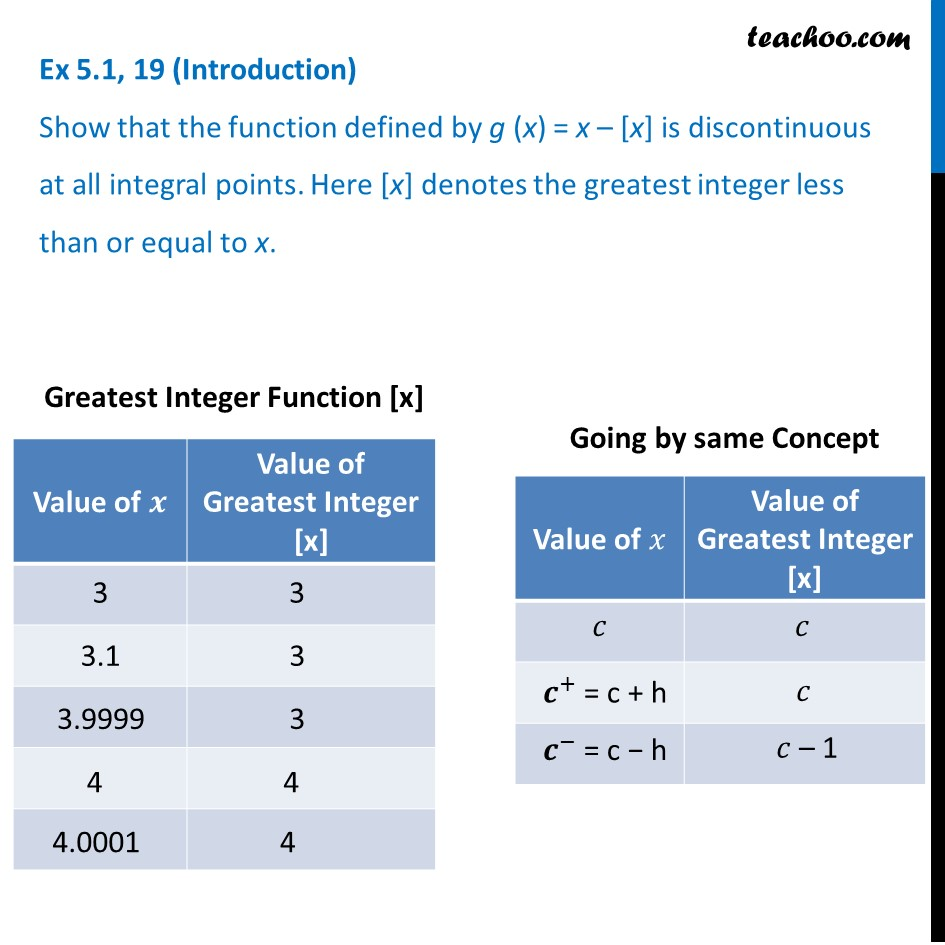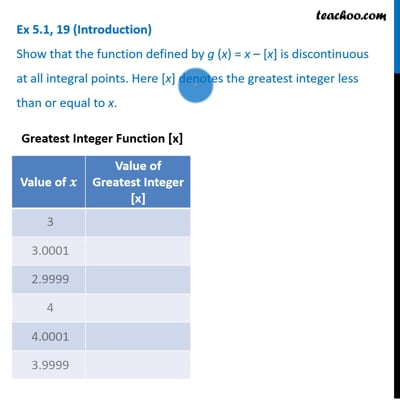This video is only available for Teachoo black users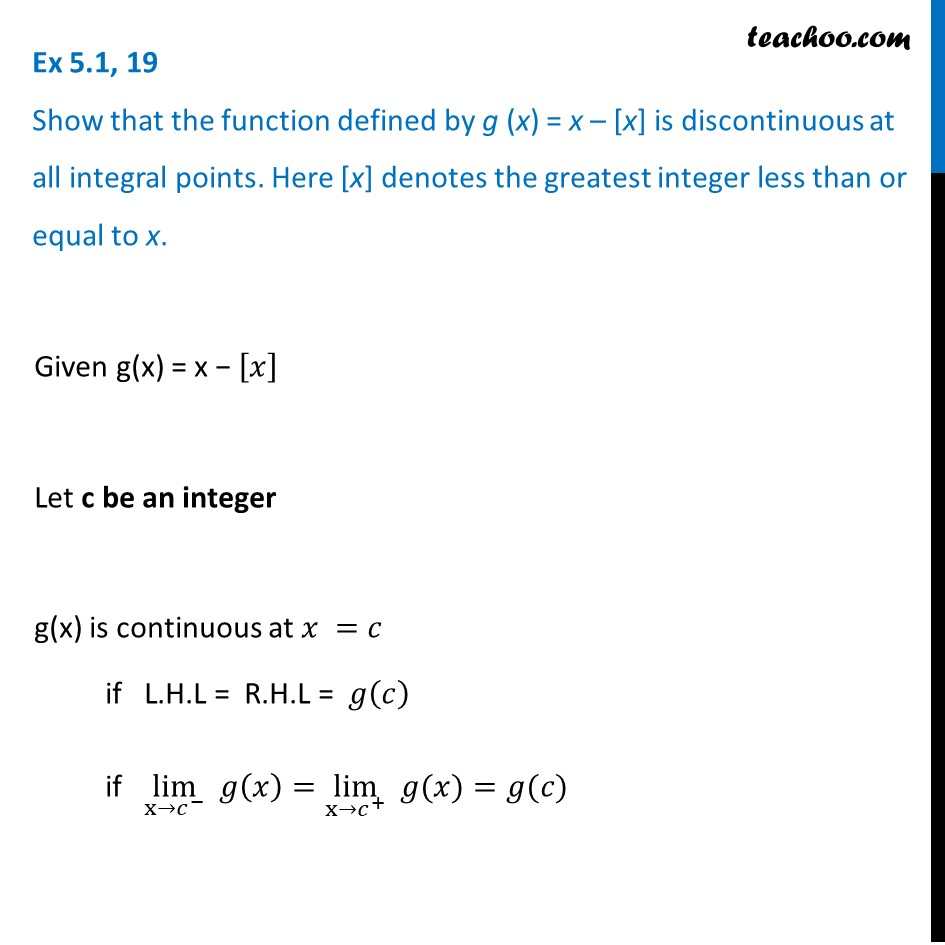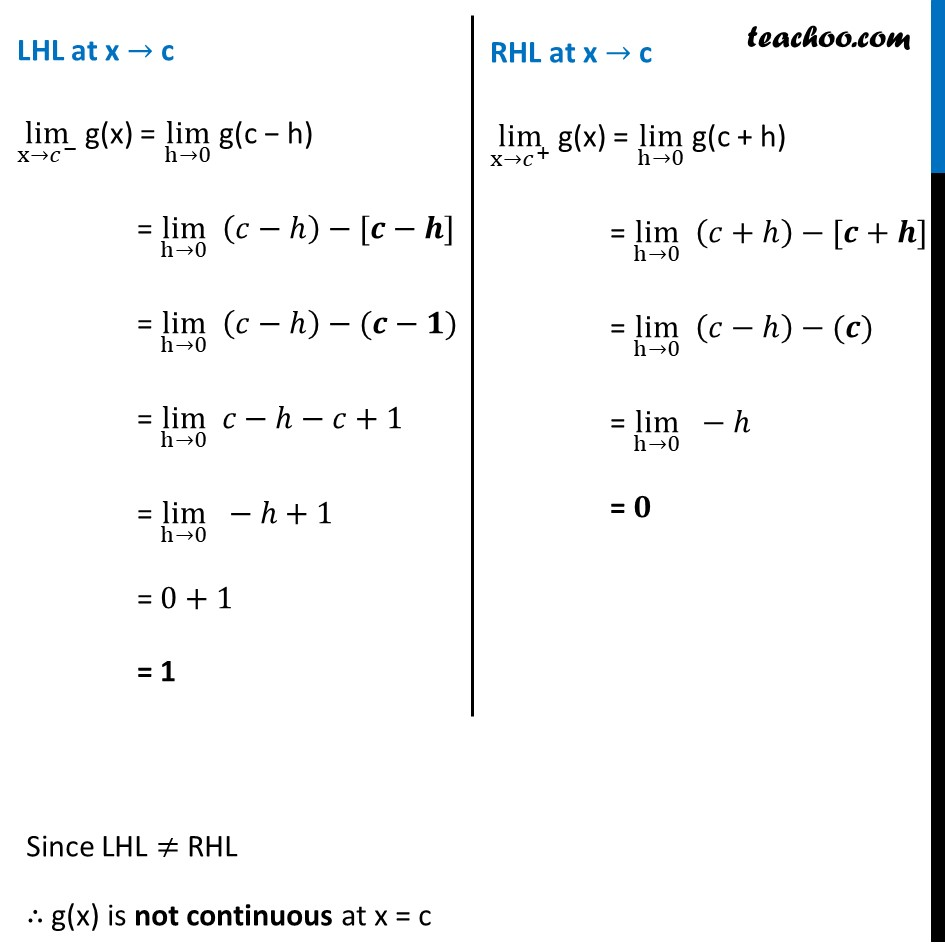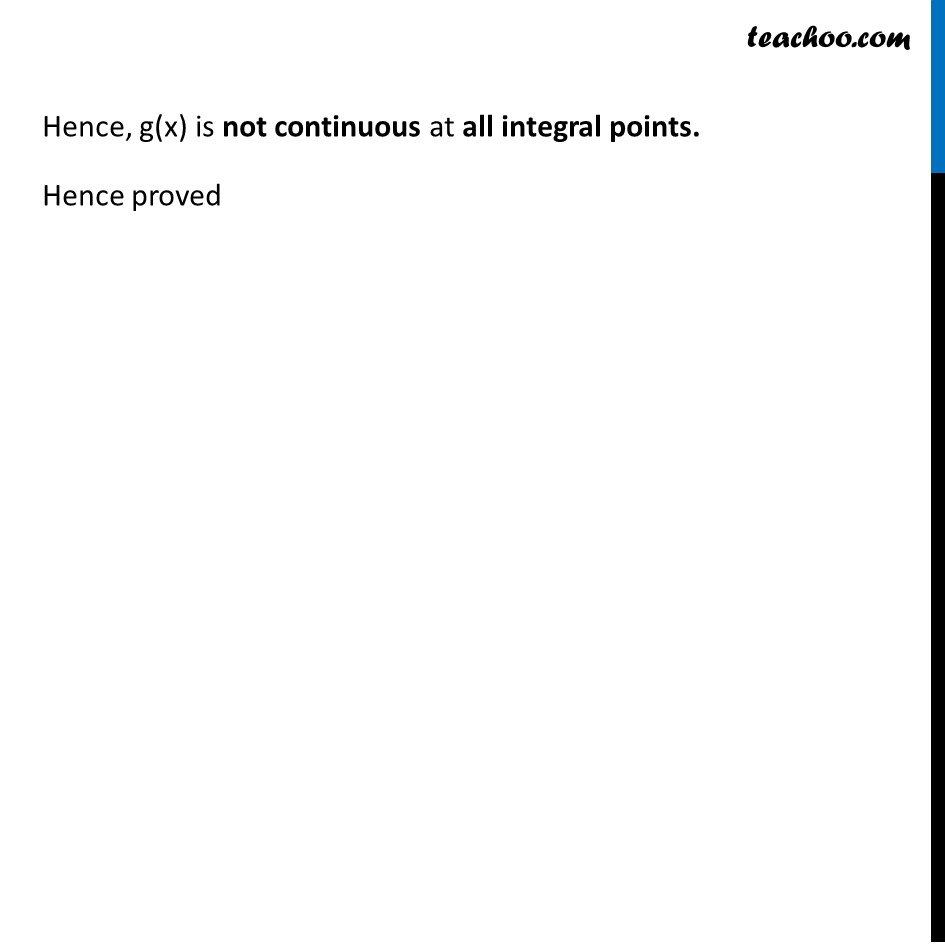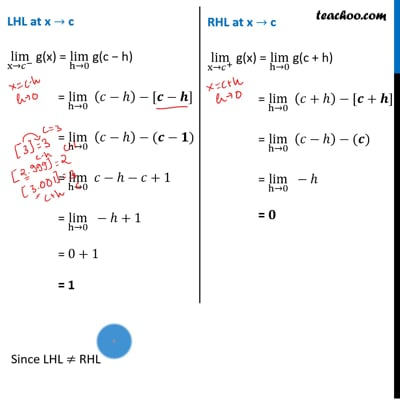This video is only available for Teachoo black users

Solve all your doubts with Teachoo Black (new monthly pack available now!)

### Transcript

Ex 5.1, 19 (Introduction) Show that the function defined by g (x) = x – [x] is discontinuous at all integral points. Here [x] denotes the greatest integer less than or equal to x. Ex 5.1, 19 Show that the function defined by g (x) = x – [x] is discontinuous at all integral points. Here [x] denotes the greatest integer less than or equal to x. Given g(x) = x − [𝑥] Let c be an integer g(x) is continuous at 𝑥 =𝑐 if L.H.L = R.H.L = 𝑔(𝑐) if lim┬(x→𝑐^− ) 𝑔(𝑥)=lim┬(x→𝑐^+ ) " " 𝑔(𝑥)=𝑔(𝑐) LHL at x → c lim┬(x→𝑐^− ) g(x) = lim┬(h→0) g(c − h) = lim┬(h→0) (𝑐−ℎ)−[𝒄−𝒉] = lim┬(h→0) (𝑐−ℎ)−(𝒄−𝟏) = lim┬(h→0) 𝑐−ℎ−𝑐+1 = lim┬(h→0) −ℎ+1 = 0+1 = 1 RHL at x → c lim┬(x→𝑐^+ ) g(x) = lim┬(h→0) g(c + h) = lim┬(h→0) (𝑐+ℎ)−[𝒄+𝒉] = lim┬(h→0) (𝑐−ℎ)−(𝒄) = lim┬(h→0) −ℎ = 𝟎 Since LHL ≠ RHL ∴ g(x) is not continuous at x = c Hence, g(x) is not continuous at all integral points. Hence proved